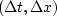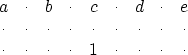Next: The prediction form of Up: 2-D INTERPOLATION BEYOND ALIASING Previous: Interpolation with spatial predictors

## Refining both t and x with a spatial predictor

Having determined a 2-D filter, say on the original data mesh, we can now interlace both t and x and expect to use the identical filter. This is because slopes are preserved if we replaceby.Everyone knows how to interpolate data on the time mesh, so that leaves the job of interpolation on the space mesh: in (6) the known (a,b,c,d,e) can multiply a known trace, and then the 1'' can multiply the interlaced and unknown trace. It is then easy to minimize the power out by defining the missing trace to be the negative of that predicted by the filter (a,b,c,d,e) on the known trace. (The spatial interpolation problem seems to be solved regardless of the amount of the signal shift. A spatial aliasing'' issue does not seem to arise.) It is nice to think of the unknowns being under the 1'' and the knowns being under the (a,b,c,d,e), but the CG method has no trouble working backwards too.

After I became accustomed to using the CG method, I stopped thinking that the unknown data is that which is predicted, and instead began to think that the unknown data is that which minimizes the power out of the prediction filter. I ignored the question of which data values are known and which are unknown. This thinking enables a reformulation of the problem, so that interpolation on the time axis is an unnecessary step. This is the way all my programs work. Think of the filter that follows as applied on the original coarse-mesh data:(7)
The first stage is to use CG to find (a,b,c,d,e) in (7). For the second stage, we assert that the same values (a,b,c,d,e) found from (7) can be used in (6), and we use CG a second time to find the missing data values. A wave field interpolated this way is shown in Figure 17. Figures 13 to 16 were made with filters that had more rows than (7), for reasons we will discuss next.lace1
Figure 17
Two signals with one dip.Next: The prediction form of Up: 2-D INTERPOLATION BEYOND ALIASING Previous: Interpolation with spatial predictors
Stanford Exploration Project
10/21/1998